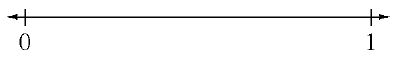### Home > CC1MN > Chapter 8 > Lesson 8.1.1 > Problem8-13

8-13.

Copy the number line and label the following numbers at their approximate place on the number line.1.  $\frac { 14 } { 28 }$

1.  $0.75$

1.  $\frac { 1 } { 3 }$

1.  $\frac { 4 } { 7 }$

$\text{Can you decide if each portion is greater than or less than }0.5\ (\text{or }\frac{1}{2})?$